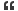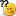# Thread: How to find submatix with max sum

1. ## How to find submatix with max sum

How to find submatix with max sumReply With Quote

2. ## Re: How to find submatix with max sumOriginally Posted by Geek_GuestHow to find submatix with max sum
Sorry....cud u ellaborate the Question..Reply With Quote

3. ## Re: How to find submatix with max sum

List all the Sub Matrices and find the sum of each matrix and then find the maximum sum.

Let us consider inputArr[m][n] be the input array.
And let us make another array outputArr[m][n] with all fields set to zero by default.
Each element of the array say outputArr[i][j] will store the sum of elements from inputArr till inputArr[i][j].

So determine outputArr[i][j] =
inputArr[i][j] + outputArr[i-1][j] + outputArr[i][j-1] - outputArr[i-1][j-1]

Now to find the matrix sum of a matrix starting at i1,j1 till i2,j2 is
sum = outputArr[i2][j2]- outputArr[i1][j2] - outputArr[i2][j1] + outputArr[i1-1][j1-1]

Now we can run an algorithm

For each sub matrix find the sum and then compare with the maximum sum found till now. Then output the result in the end.Reply With Quote

####Posting Permissions

• You may not post new threads
• You may not post replies
• You may not post attachments
• You may not edit your posts
•